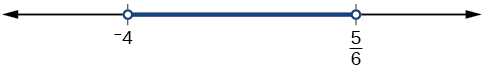## Understanding Compound Inequalities

A compound inequality includes two inequalities in one statement. A statement such as $4<x\le 6$ means $4<x$ and $x\le 6$. There are two ways to solve compound inequalities: separating them into two separate inequalities or leaving the compound inequality intact and performing operations on all three parts at the same time. We will illustrate both methods.

### Example 7: Solving a Compound Inequality

Solve the compound inequality: $3\le 2x+2<6$.

### Solution

The first method is to write two separate inequalities: $3\le 2x+2$ and $2x+2<6$. We solve them independently.

$\begin{array}{lll}3\le 2x+2\hfill & \text{and}\hfill & 2x+2<6\hfill \\ 1\le 2x\hfill & \hfill & 2x<4\hfill \\ \frac{1}{2}\le x\hfill & \hfill & x<2\hfill \end{array}$

Then, we can rewrite the solution as a compound inequality, the same way the problem began.

$\frac{1}{2}\le x<2$

In interval notation, the solution is written as $\left[\frac{1}{2},2\right)$.

The second method is to leave the compound inequality intact, and perform solving procedures on the three parts at the same time.

$\begin{array}{ll}3\le 2x+2<6\hfill & \hfill \\ 1\le 2x<4\hfill & \text{Isolate the variable term, and subtract 2 from all three parts}.\hfill \\ \frac{1}{2}\le x<2\hfill & \text{Divide through all three parts by 2}.\hfill \end{array}$

We get the same solution: $\left[\frac{1}{2},2\right)$.

### Try It 7

Solve the compound inequality $4<2x - 8\le 10$.

Solution

### Example 8: Solving a Compound Inequality with the Variable in All Three Parts

Solve the compound inequality with variables in all three parts: $3+x>7x - 2>5x - 10$.

### Solution

Lets try the first method. Write two inequalities:

$\begin{array}{lll}3+x> 7x - 2\hfill & \text{and}\hfill & 7x - 2> 5x - 10\hfill \\ 3> 6x - 2\hfill & \hfill & 2x - 2> -10\hfill \\ 5> 6x\hfill & \hfill & 2x> -8\hfill \\ \frac{5}{6}> x\hfill & \hfill & x> -4\hfill \\ x< \frac{5}{6}\hfill & \hfill & -4< x\hfill \end{array}$

The solution set is $-4<x<\frac{5}{6}$ or in interval notation $\left(-4,\frac{5}{6}\right)$. Notice that when we write the solution in interval notation, the smaller number comes first. We read intervals from left to right, as they appear on a number line.Figure 3

### Try It 8

Solve the compound inequality: $3y<4 - 5y<5+3y$.

Solution